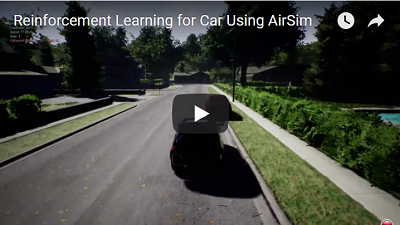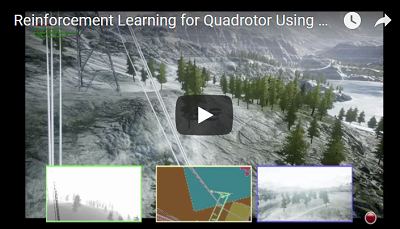# Reinforcement Learning in AirSim#

We below describe how we can implement DQN in AirSim using an OpenAI gym wrapper around AirSim API, and using stable baselines implementations of standard RL algorithms. We recommend installing stable-baselines3 in order to run these examples (please see https://github.com/DLR-RM/stable-baselines3)

#### Disclaimer#

This is still in active development. What we share below is a framework that can be extended and tweaked to obtain better performance.

#### Gym wrapper#

In order to use AirSim as a gym environment, we extend and reimplement the base methods such as step, _get_obs, _compute_reward and reset specific to AirSim and the task of interest. The sample environments used in these examples for car and drone can be seen in PythonClient/reinforcement_learning/*_env.py

## RL with Car#

Source code

This example works with AirSimNeighborhood environment available in releases.

First, we need to get the images from simulation and transform them appropriately. Below, we show how a depth image can be obtained from the ego camera and transformed to an 84X84 input to the network. (you can use other sensor modalities, and sensor inputs as well – of course you’ll have to modify the code accordingly).

responses = client.simGetImages([ImageRequest(0, AirSimImageType.DepthPerspective, True, False)])
current_state = transform_input(responses)

We further define the six actions (brake, straight with throttle, full-left with throttle, full-right with throttle, half-left with throttle, half-right with throttle) that an agent can execute. This is done via the function interpret_action:

def interpret_action(action):
car_controls.brake = 0
car_controls.throttle = 1
if action == 0:
car_controls.throttle = 0
car_controls.brake = 1
elif action == 1:
car_controls.steering = 0
elif action == 2:
car_controls.steering = 0.5
elif action == 3:
car_controls.steering = -0.5
elif action == 4:
car_controls.steering = 0.25
else:
car_controls.steering = -0.25
return car_controls

We then define the reward function in _compute_reward as a convex combination of how fast the vehicle is travelling and how much it deviates from the center line. The agent gets a high reward when its moving fast and staying in the center of the lane.

def _compute_reward(car_state):
MAX_SPEED = 300
MIN_SPEED = 10
thresh_dist = 3.5
beta = 3

z = 0
pts = [np.array([0, -1, z]), np.array([130, -1, z]), np.array([130, 125, z]), np.array([0, 125, z]), np.array([0, -1, z]), np.array([130, -1, z]), np.array([130, -128, z]), np.array([0, -128, z]), np.array([0, -1, z])]
pd = car_state.position
car_pt = np.array(list(pd.values()))

dist = 10000000
for i in range(0, len(pts)-1):
dist = min(dist, np.linalg.norm(np.cross((car_pt - pts[i]), (car_pt - pts[i+1])))/np.linalg.norm(pts[i]-pts[i+1]))

#print(dist)
if dist > thresh_dist:
reward = -3
else:
reward_dist = (math.exp(-beta*dist) - 0.5)
reward_speed = (((car_state.speed - MIN_SPEED)/(MAX_SPEED - MIN_SPEED)) - 0.5)
reward = reward_dist + reward_speed

return reward

The compute reward function also subsequently determines if the episode has terminated (e.g. due to collision). We look at the speed of the vehicle and if it is less than a threshold than the episode is considered to be terminated.

done = 0
if reward < -1:
done = 1
if car_controls.brake == 0:
if car_state.speed <= 5:
done = 1
return done

The main loop then sequences through obtaining the image, computing the action to take according to the current policy, getting a reward and so forth. If the episode terminates then we reset the vehicle to the original state via reset():

client.reset()
client.enableApiControl(True)
client.armDisarm(True)
car_control = interpret_action(1) // Reset position and drive straight for one second
client.setCarControls(car_control)
time.sleep(1)

Once the gym-styled environment wrapper is defined as in car_env.py, we then make use of stable-baselines3 to run a DQN training loop. The DQN training can be configured as follows, seen in dqn_car.py.

model = DQN(
"CnnPolicy",
env,
learning_rate=0.00025,
verbose=1,
batch_size=32,
train_freq=4,
target_update_interval=10000,
learning_starts=200000,
buffer_size=500000,
exploration_fraction=0.1,
exploration_final_eps=0.01,
device="cuda",
tensorboard_log="./tb_logs/",
)

A training environment and an evaluation envrionment (see EvalCallback in dqn_car.py) can be defined. The evaluation environoment can be different from training, with different termination conditions/scene configuration. A tensorboard log directory is also defined as part of the DQN parameters. Finally, model.learn() starts the DQN training loop. Similarly, implementations of PPO, A3C etc. can be used from stable-baselines3.

Note that the simulation needs to be up and running before you execute dqn_car.py. The video below shows first few episodes of DQN training.Source code

This example works with AirSimMountainLandscape environment available in releases.

We can similarly apply RL for various autonomous flight scenarios with quadrotors. Below is an example on how RL could be used to train quadrotors to follow high tension power lines (e.g. application for energy infrastructure inspection). There are seven discrete actions here that correspond to different directions in which the quadrotor can move in (six directions + one hovering action).

def interpret_action(self, action):
if action == 0:
elif action == 1:
elif action == 2:
elif action == 3:
elif action == 4:
elif action == 5:
else:
quad_offset = (0, 0, 0)

The reward again is a function how how fast the quad travels in conjunction with how far it gets from the known powerlines.

def compute_reward(quad_state, quad_vel, collision_info):
thresh_dist = 7
beta = 1

z = -10
pts = [np.array([-0.55265, -31.9786, -19.0225]),np.array([48.59735, -63.3286, -60.07256]),np.array([193.5974, -55.0786, -46.32256]),np.array([369.2474, 35.32137, -62.5725]),np.array([541.3474, 143.6714, -32.07256]),]

if self.state["collision"]:
reward = -100
else:
dist = 10000000
for i in range(0, len(pts) - 1):
dist = min(dist, np.linalg.norm(np.cross((quad_pt - pts[i]), (quad_pt - pts[i + 1]))) / np.linalg.norm(pts[i] - pts[i + 1]))

if dist > thresh_dist:
reward = -10
else:
reward_dist = math.exp(-beta * dist) - 0.5
reward_speed = (np.linalg.norm([self.state["velocity"].x_val, self.state["velocity"].y_val, self.state["velocity"].z_val,])- 0.5)
reward = reward_dist + reward_speed

We consider an episode to terminate if it drifts too much away from the known power line coordinates, and then reset the drone to its starting point.

Once the gym-styled environment wrapper is defined as in drone_env.py, we then make use of stable-baselines3 to run a DQN training loop. The DQN training can be configured as follows, seen in dqn_drone.py.

model = DQN(
"CnnPolicy",
env,
learning_rate=0.00025,
verbose=1,
batch_size=32,
train_freq=4,
target_update_interval=10000,
learning_starts=10000,
buffer_size=500000,
)
A training environment and an evaluation envrionment (see EvalCallback in dqn_drone.py) can be defined. The evaluation environoment can be different from training, with different termination conditions/scene configuration. A tensorboard log directory is also defined as part of the DQN parameters. Finally, model.learn() starts the DQN training loop. Similarly, implementations of PPO, A3C etc. can be used from stable-baselines3.